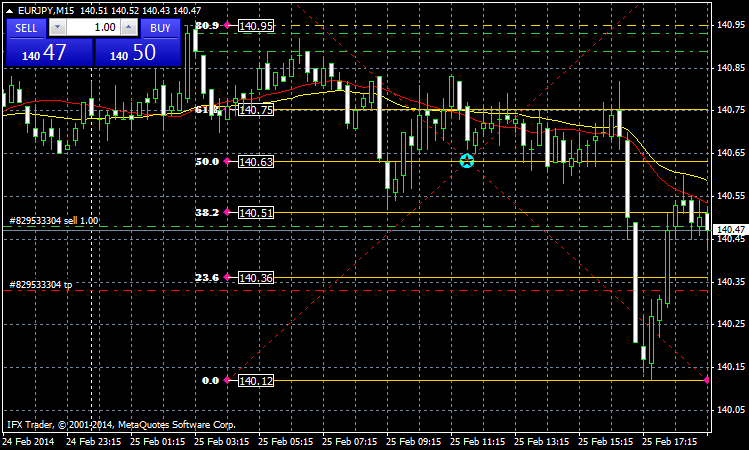# FiboRetracementLevels – Indicator For MetaTrader 4FiboRetracementLevels – Indicator For MetaTrader 4 free indicator for day trading. Fibonacci Retracement Levels indicator draws Fibonacci Retracement Levels on the trading chart

The Fibonacci retracements pattern can be useful for swing traders to identify reversals on a stock chart. On this page we will look at the Fibonacci sequence and show some examples of how you can identify this pattern.

Fibonacci numbers were developed by Leonardo Fibonacci and it is simply a series of numbers that when you add the previous two numbers you come up with the next number in the sequence. Here is an example:

1, 2, 3, 5, 8, 13, 21, 34, 55

### Description:

Fibonacci Retracement Levels indicator draws Fibonacci Retracement Levels on the trading chart.

Version 2: added price labels on each Fibonacci level.